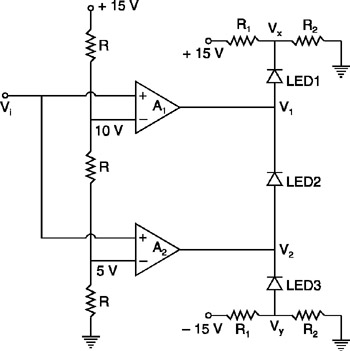The Linear and Digital Integrated Circuits Design Primer

# 4.11: WINDOW DETECTOR

## 4.11 WINDOW DETECTOR

A window detector is a special type of comparator. In this case, many comparators will be working in parallel, with different reference voltages. By noting the outputs from the different comparators, the range which contains the input can be noted.

A typical window detector circuit is shown in Figure 4.30.Figure 4.30: Window detector circuit.

In this example, two non-inverting comparators are used. The total voltage, 15 V, is divided into three equal voltage steps, with the help of three equal resistances R. Therefore, the voltage above one resistance will be + 5 V and the voltage above two resistances will be + 10 V.

V x can be calculated from R 1 and R 2 and can be noted as a positive voltage. Similarly V y can be noted as a negative voltage.

If input V i is less than 5 V, A 2 produces a output of ? V sat and A 1 also produces a output of ? V sat. In this state LED3 is forward biased and LED2 is not forward biased to the sufficient extent, and LED1 is reverse biased. Since forward biased LEDs will emit light, LED3 will glow and the other LEDs will not.

If input V i is between 5 V and 10 V, A 2 produces an output of + V sat and A 1 produces an output of ? V sat. Under...### This is embarrasing...

An error occurred while processing the form. Please try again in a few minutes.